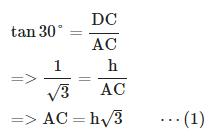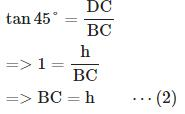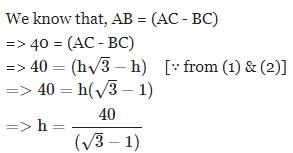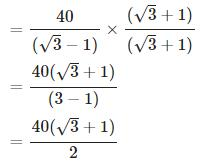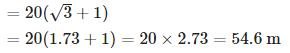Courses

# Test: Heights And Distances - 1

## 10 Questions MCQ Test Quantitative Aptitude for GMAT | Test: Heights And Distances - 1

Description
This mock test of Test: Heights And Distances - 1 for GMAT helps you for every GMAT entrance exam. This contains 10 Multiple Choice Questions for GMAT Test: Heights And Distances - 1 (mcq) to study with solutions a complete question bank. The solved questions answers in this Test: Heights And Distances - 1 quiz give you a good mix of easy questions and tough questions. GMAT students definitely take this Test: Heights And Distances - 1 exercise for a better result in the exam. You can find other Test: Heights And Distances - 1 extra questions, long questions & short questions for GMAT on EduRev as well by searching above.
QUESTION: 1

### Two ships are sailing in the sea on the two sides of a lighthouse. The angle of elevation of the top of the lighthouse is observed from the ships are 30º and 45º respectively. If the lighthouse is 100 m high, the distance between the two ships is:

Solution: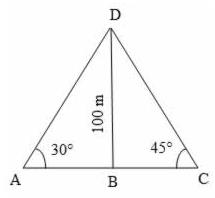Let BD be the lighthouse and A and C be the positions of the ships.
Then, BD = 100 m,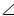BAD = 30° ,BCD = 45°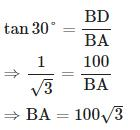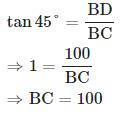Distance between the two ships
= AC = BA + BC
=100√3+100
=100(√3+1)
= 100(1.73+1) = 100 × 2.73 = 273 m

QUESTION: 2

### A man standing at a point P is watching the top of a tower, which makes an angle of elevation of 30º with the man's eye. The man walks some distance towards the tower to watch its top and the angle of the elevation becomes 45º. What is the distance between the base of the tower and the point P?

Solution:

One of AB, AD and CD must have given.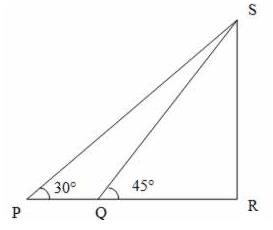QUESTION: 3

### From a point P on a level ground, the angle of elevation of the top tower is 30º. If the tower is 200 m high, the distance of point P from the foot of the tower is:

Solution: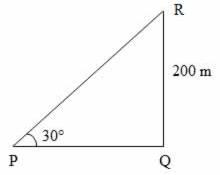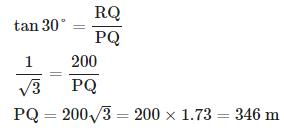QUESTION: 4

The angle of elevation of the sun, when the length of the shadow of a tree is equal to the height of the tree, is:

Solution: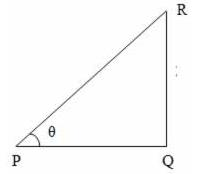Consider the diagram shown above where QR represents the tree and PQ represents its shadow

We have, QR = PQ
Let angleQPR = θ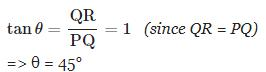i.e., required angle of elevation = 45°

QUESTION: 5

An observer 2 m tall is 10√3103 m away from a tower. The angle of elevation from his eye to the top of the tower is 30º. The height of the tower is:

Solution: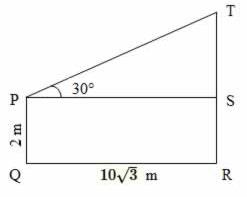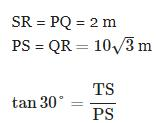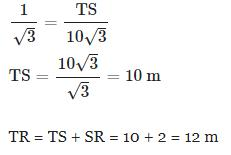QUESTION: 6

The angle of elevation of a ladder leaning against a wall is 60º and the foot of the ladder is 12.4 m away from the wall. The length of the ladder is:

Solution: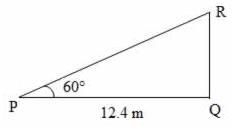Consider the diagram shown above where PR represents the ladder and RQ represents the wall.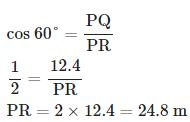QUESTION: 7

A man on the top of a vertical observation tower observers a car moving at a uniform speed coming directly towards it. If it takes 8 minutes for the angle of depression to change from 30° to 45°, how soon after this will the car reach the observation tower?

Solution: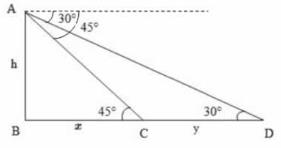Consider the diagram shown above. Let AB be the tower. Let D and C be the positions of the car.

Then, ∠ADC = 30° , ∠ACB = 45°

Let AB = h, BC = x, CD = y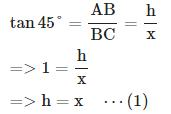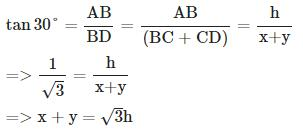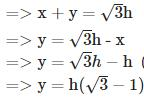Given that distance y is covered in 8 minutes.
i.e, distance h(√3−1) is covered in 8 minutes.
Time to travel distance x
= Time to travel distance h (∵ Since x = h as per equation 1).

Let distance h is covered in t minutes.

since distance is proportional to the time when the speed is constant, we have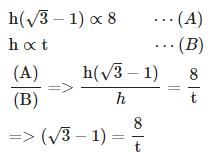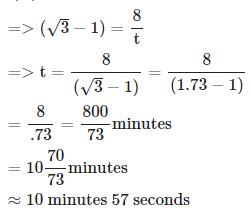QUESTION: 8

A man is watching from the top of a tower a boat speeding away from the tower. The boat makes an angle of depression of 45° with the man's eye when at a distance of 100 metres from the tower. After 10 seconds, the angle of depression becomes 30°. What is the approximate speed of the boat, assuming that it is running in still water?

Solution: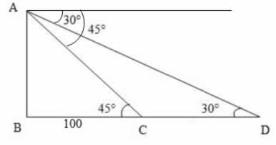Consider the diagram shown above.

Let AB be the tower. Let C and D be the positions of the boat

Then,ACB = 45° ,ADC = 30°, BC = 100 m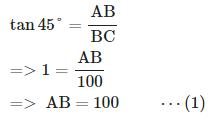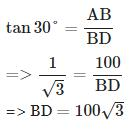CD = (BD - BC) =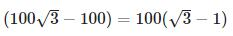It is given that the distance CD is covered in 10 seconds.
i.e., the distance 100(√3−1) is covered in 10 seconds.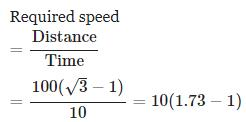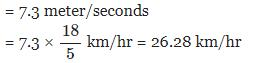QUESTION: 9

The top of a 15 metre high tower makes an angle of elevation of 60° with the bottom of an electronic pole and angle of elevation of 30° with the top of the pole. What is the height of the electric pole?

Solution: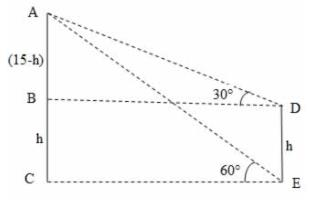Consider the diagram shown above. AC represents the tower and DE represents the pole.

Given that AC = 15 m,ADB = 30°,AEC = 60°

Let DE = h
Then, BC = DE = h,
AB = (15-h)    (∵ AC=15 and BC = h),
BD = CE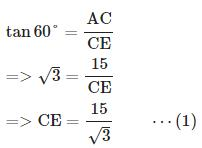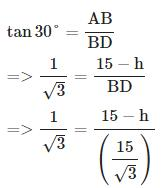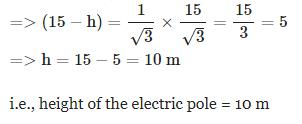QUESTION: 10

The angle of elevation of the top of a tower from a certain point is 30°. If the observer moves 40 m towards the tower, the angle of elevation of the top of the tower increases by 15°. The height of the tower is:

Solution: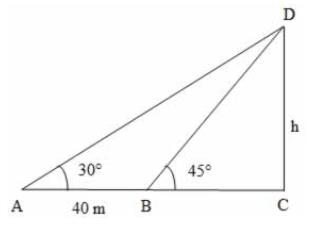Let DC be the tower and A and B be the positions of the observer such that AB = 40 m

We haveDAC = 30°,DBC = 45°

Let DC = h